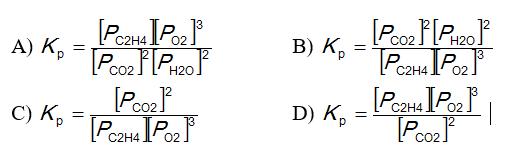Chemistry Practice Problems Equilibrium Expressions Practice Problems Solution: What is the equilibrium equation for the following...

🤓 Based on our data, we think this question is relevant for Professor Casselman's class at UCR.

# Solution: What is the equilibrium equation for the following reaction?C 2 H 4 (g) + 3 O 2 (g) ⇌ 2 CO 2 (g) + 2 H 2 O (l)

###### Problem

What is the equilibrium equation for the following reaction?

C 2 H 4 (g) + 3 O 2 (g) ⇌ 2 CO 2 (g) + 2 H 2 O (l)Equilibrium Expressions

Equilibrium Expressions

#### Q. On analysis, an equilibrium mixture for the reaction. 2H2S(g) ⇌ 2H2(g) + S2(g)was found to contain 1.0 mol H2S, 4.0 mol H2, and 0.80 mol S2 in a 4.0 L...

Solved • Mon Mar 06 2017 09:27:11 GMT-0500 (EST)

Equilibrium Expressions

#### Q. Consider the reaction C (s) + CO2 (g) → 2 CO(g) .At equilibrium at a certain temperature, the partial pressures of CO(g) and CO2(g) are 1.22 atm and 0...

Solved • Fri Feb 24 2017 09:25:10 GMT-0500 (EST)

Equilibrium Expressions

#### Q. The  equilibrium constant for the reaction                               2 H   2 (g)  +   CO (g) ⇌ CH 3OH (g) is 1.6 x 10-8 at a certain temperature. ...

Solved • Fri Feb 17 2017 11:03:26 GMT-0500 (EST)

Equilibrium Expressions

#### Q. A mixture of N2 (g) and H2 (g) attained equilibrium at 745 K in the reaction: N2 (g) + 3H2 (g) ⇌ 2NH3 (g). The equilibrium mixture of gases was anal...

Solved • Mon Oct 17 2016 17:27:36 GMT-0400 (EDT)